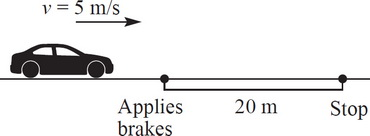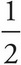# AP Physics 1 Question 286: Answer and Explanation

### Test Information

Question: 2864. A car of mass 1000 kg is traveling at a speed of 5 m/s. The driver applies the breaks, generating a constant friction force, and skids for a distance of 20 m before coming to a complete stop. Given this information, what is the coefficient of friction between the car's tires and the ground?

• A. 0.25
• B. 0.2
• C. 0.125
• D. 0.0625

First, you know Ff = μFN = µ(mg). Second, you know that W = Δ = KEf - KE0 = -KE0 (since KEf = 0 in this case) = -mv02. Plugging in numbers, you get W = -(1000 kg)(5 m/s)2 = -12,500 J. You also know that W = Fdcosθ = [µ(mg)]dcosθ. Solving for μ, you get μ = W/[(mg)dcosθ]. Plugging in the numbers then gives μ = -12,500 J/[(1000 kg)(10 m/s2)(20 m)(cos180°)] = 0.0625.